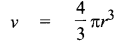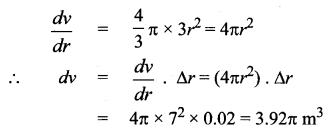## Tamilnadu Samacheer Kalvi 12th Maths Solutions Chapter 8 Differentials and Partial Derivatives Ex 8.1

Question 1.
Let f(x) = $$\sqrt{x}$$. Find the linear approximation at x = 27. Use the linear approximation to $$\sqrt{27.2}$$.
Solution: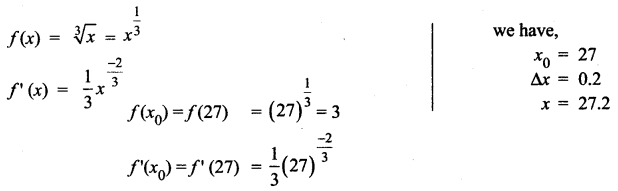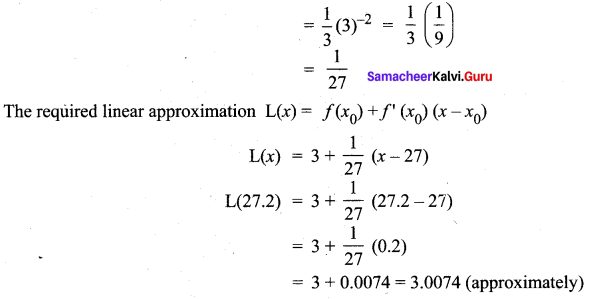Question 2.
Use the linear approximation to find approximate values of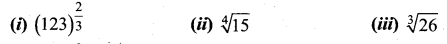Solution: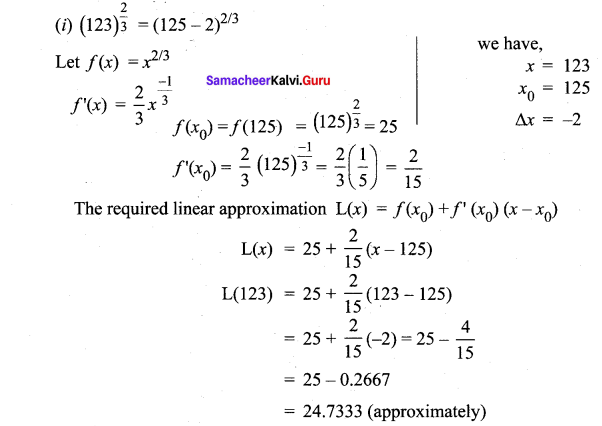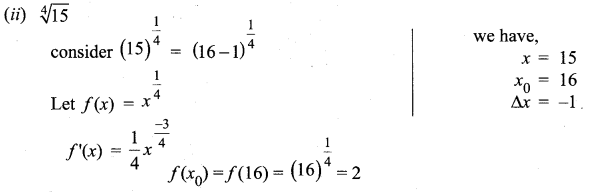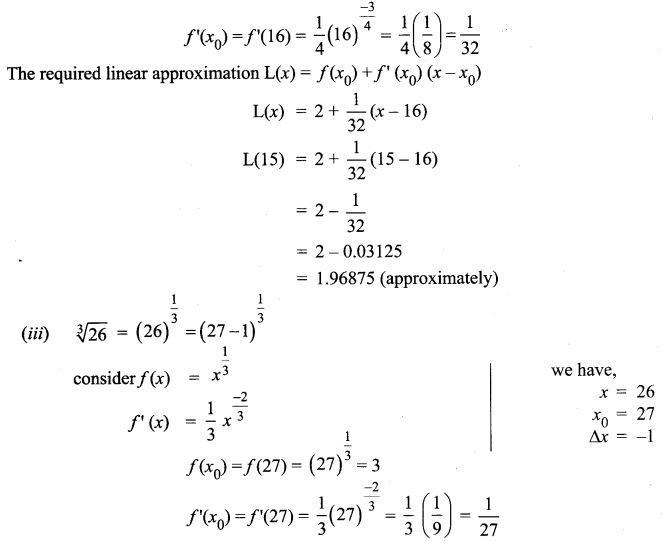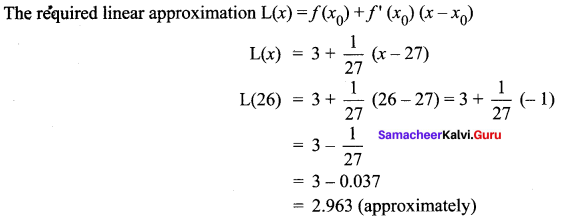Question 3.
Find a linear approximation for the following functions at the indicated points.
(i) f(x) = x3 – 5x + 12, x0 = 2
(ii) g(x) = $$\sqrt{x^{2}+9}$$ + x0 = -4
(iii) h(x) = $$\frac{x}{x+1}$$, x0 = 1
Solution:
f(x) = x3 – 5x + 12
f'(x) = 3x2 – 5
f(x0) = f(2) = (2)3 – 5(2) + 12 = 8 – 10 + 12 = 10
f'(x0) = f'(2) = 3(2)2 – 5 = 12 – 5 = 7
The required linear approximation L(x) = f(x0) + f'(x0) (x – x0)
= 10 + 7 (x – 2)
= 10 + 7x – 14
= 7x – 4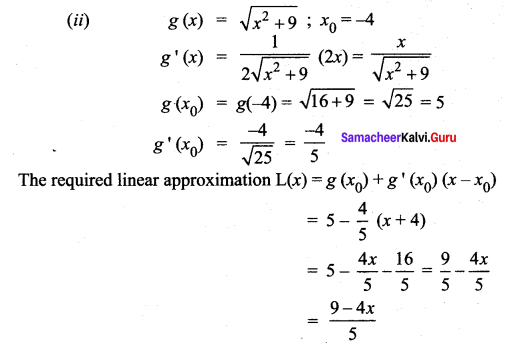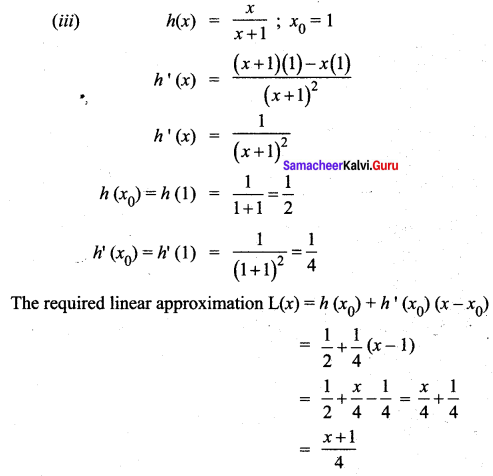Question 4.
The radius of a circular plate is measured as 12.65 cm instead of the actual length 12.5 cm. find the following in calculating the area of the circular plate:
(i) Absolute error
(ii) Relative error
(iii) Percentage error
Solution:
We know that Area of the circular plate A(r) = πr2, A'(r) = 2πr
Change in Area = A’ (12.5) (0.15) = 3.75 π cm2
Exact calculation of the change in Area = A (12.65) – A (12.5)
= 160.0225π – 156.25π
= 3.7725π cm2
(i) Absolute error = Actual value – Approximate value
= 3.7725 π – 3.75 π
= 0.0225 π cm2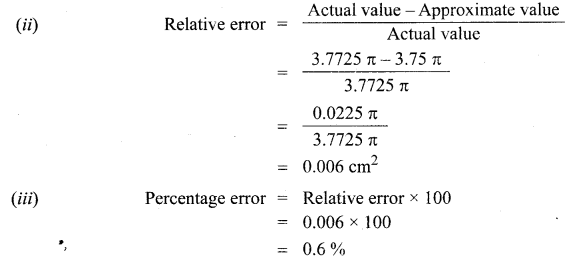Question 5.
A sphere is made of ice having radius 10 cm. Its radius decreases from 10 cm to 9.8 cm. Find approximations for the following:
(i) change in the volume
(ii) change in the surface area
Solution:
(i) We know that Volume of sphere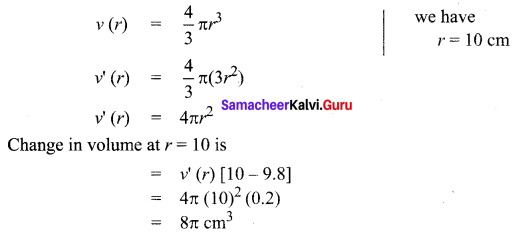∴ Volume decreases by 80π cm3

(ii) Surface area of the sphere
S(r) = 4π r2
S'(r) = 8πr
Change in surface area at r = 10 is
= S'(r) [10 – 9.8]
= 8π (10) (0.2) = 16π cm2
∴ Surface Area decreases by 16π cm2

Question 6.
The time T, taken for a complete oscillation of a single pendulum with length l, is given
by the equation T = $$2 \pi \sqrt{\frac{l}{g}}$$, where g is a constant. Find the approximate percentage error in the calculated value of T corresponding to an error of 2 percent in the value of 1.
Solution: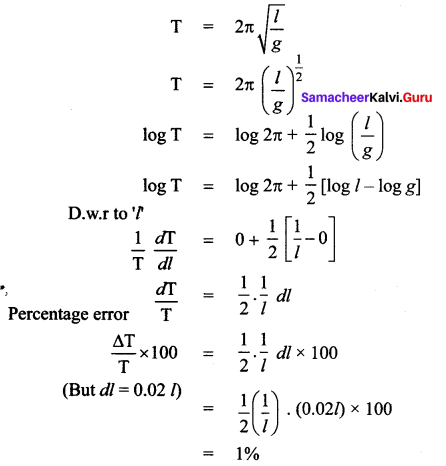Question 7.
Show that the percentage error in the nth root of a number is approximately $$\frac{1}{n}$$ times the percentage error in the number.
Solution:
Let x be the number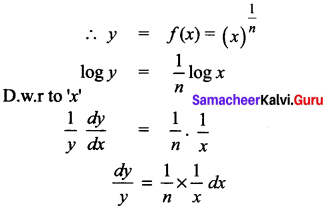### Samacheer Kalvi 12th Maths Solutions Chapter 8 Differentials and Partial Derivatives Ex 8.1 Additional Questions Solved

Question 1.
Using differentials, find the approximate value of each of the following upto 3 places of decimal. $$(255)^{\frac{1}{4}}$$
Solution: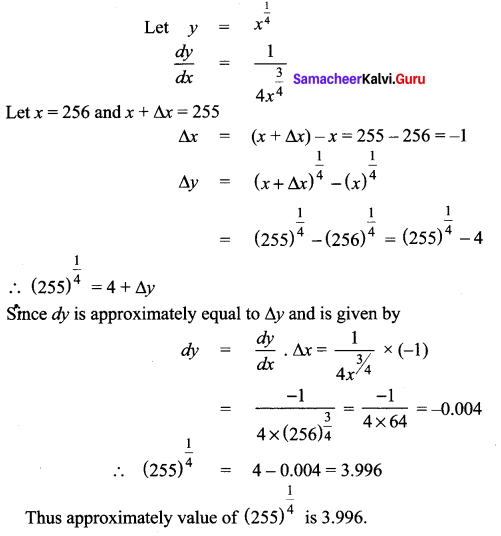Question 2.
Using differentials, find the approximate value of each of the following upto 3 places of decimal. $$(401)^{\frac{1}{2}}$$
Solution: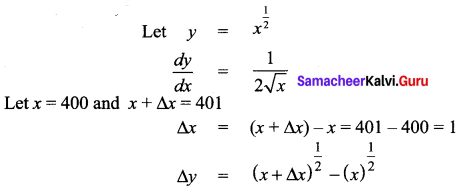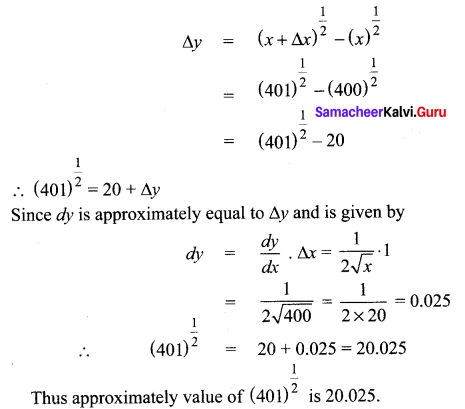Question 3.
Find approximate value of f(5.001) where f(x) = x3 – 7x2 + 15.
Solution:
Here f(x) = x3 – 7x2 + 15
f'(x) = 3x2 – 14x
Let x = 5 and x + ∆x = 5.001
∴ ∆x (x + ∆x) – x = 5.001 – 5
= 0.001
f(5.001) = f(x + ∆x)
Now ∆y = f(x + ∆x) – f(x)
f(x + ∆x) = f(x) + ∆y
= f(x) + f'(x).∆x [∴ ∆y = f'(x).∆x ]
= (x3 – 7x2 + 15) + (3x2 – 14x)(0.001)
= (53 – 7 × 52 + 15) + (3 × 52 – 14 × 5) [0.001]
= [125 – 175 + 15] + [75 – 70][0.001]
= -35 + 0.005 = – 34.995
Thus approximate value of f(5.001) is – 34.995Question 4.
If the radius of a sphere is measured as 7m with an error of 0.02 m then find the approximate error in calculating its volume.
Solution:
Le r be the radius of the sphere and ∆r be the error in measuring the radius.
Then r = 7 m and ∆r = 0.02 m
Now volume of a sphere is given by